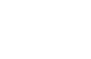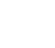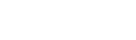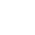## Mathematics P1 Questions and Answers - Butula Sub-County Post Mock Exams 2021/2022

Instructions to candidates.

• Write your name and index number in the spaces provided above.
• Sign and write the date of the examination in spaces provided above.
• This paper consists of two sections: Section I and II.
• Answer all the questions in section I and only five questions from section II.
• Show all the steps in your calculations, giving your answer at each stage in the space provided.
• Marks may be given for correct working even if the answer is wrong.
• Non-programmable silent electronic calculators and KNEC mathematical tables may be used, except where stated otherwise.
• Candidates should answer the questions in English.

For examiner’s use only
SECTION I

 1 2 3 4 5 6 7 8 9 10 11 12 13 14 15 16 TOTAL

SECTION II

 17 18 19 20 21 22 23 24 TOTAL## QUESTIONS

SECTION I (50 MARKS):
Answer all the questions in this section in the spaces provided.

1. Without using a calculator evaluate.(3 marks)
2. The distance between Jane’s home and her school is 4/5 of 8km. One day she run ¼ of the way and walked the rest of the journey. What distance did she walk? (3marks)
3. Otiende works for a coffee processing company as a sales man. He is paid on Monthly basis as per agreement below.
1. A basic pay of sh. 20,000 per Month.
2. A commission of 2% for goods sold up to a maximum of sh. 200,000.
3. A commission of 4% for goods sold over sh. 200,000 in that Month.
In a certain Month he sold goods worthy sh. 600,000. Calculate his total pay for that Month. (3marks)
4. The figure below is a triangular prism of uniform cross-section in which AF = FB =3cm, AB = 4cm and BC = 5cm. Draw a clearly labeled net of the prism. (3marks)5. Solve for y in the equation. 8(y+1)-2(3y+1)=48 (3marks)
6. Simplify the expression;(3marks)
7. A line P whose equation is y = 1⁄3 x + 4 is parallel to another line Q. Find the equation of line Q in the form y= mx + c given that it passes through Point ( 3, 6) (3marks)
8. The figure below shows a triangle ABC in which AB = 6cm, BC = 11cm and angle ABC = 100º. Calculate to the decimal places the length of AC. (3marks)9. A football match between Bercelona FC and Liverpoo FC started at 1500hrs. It lasted for the official 90 minutes with a half time break of 15 minutes. The referee added five extra minutes for injuries and other stoppages. Find the time the match ended. (3marks)
10. Find the region defined by the following inequalities (3marks)
2y < x + 4; 4y ≥ -x – 4; x ≤ 211. The GCD three numbers is 6 and their LCM is 900. If two of the numbers are 36 and 60, find the least possible third number. (3 marks)
12. The mass of two similar cans is 960g and 15000g. If the total surface area of the smaller can is 144cm2, determine the surface area of the larger can. (3 marks)
13. The width of a rectangular hall of Busiada Girls Secondary School is 16m less than its length. Calculate the length of the hall if its area is 32m2. Hence calculate its perimeter. (4marks)
14. Town A is 80km due east of town B. Town C is on a bearing of 234º form town B. If town C is 100km from town A, by scale drawing find the distance of town C from town B. (4marks)
15.
1. Find the inverse of the matrix. (1mark)
2. Using matrix method, solve the simultaneous equations. (2 marks)
7x + 4y = 14
3x + 2y = 8
16. Use tables of square roots and reciprocals to find the value of x. (3marks)SECTION II (50 marks).
Answer only five questions in this section in the spaces provided.

1. The figure below shows a frustrum. The top and bottom radii are 5cm and 10cm respectively, while the vertical height of the frustrum is 12cm.Find the:-
1. Slant height of the frustum. (3marks)
2. Curved area of the frustum. (3marks)
3. Volume of the frustum. (4marks)
2. Bumala is a market centre 600km from Kisumu town.A bus starts from Kisumu for Bumala at 7.00am at an average speed of 80 km/h. At 8.30 am a car started from Kisumu to Bumala and moved at an average speed of 120 km/hr. Calculate
1. The distance bus covered before the car started moving. (3marks)
2. The relative speed for the two vehicles. (2marks)
3. The time the car overtook the bus. (1 mark)
4. Distance covered by the car before overtaking the bus. (2marks)
5. Distance from Bumala to the car at the time the car was overtaking the bus. (2marks)
3. The height of 36 students in a class was recorded to the nearest centimeter as follows:-
148 159 158 163 166 155 155 179 158
161 160 157 165 165 175 173 172 178
147 168 157 172 165 154 170 157 167
155 159 173 171 168 160 172 156 167
1. Make a frequency distribution table using a class interval of 5 and starting with the class 145 – 149. (2marks)
2. From the table above
1. Calculate the mean mark (3marks)
2. Calculate the median (3marks)
3.
4. Draw a frequency polygon using the table in (a) above. (2 marks)4. Bujumba Boys Secondary School. Intends to buy a certain number of chairs For Ksh. 16,200. The supplier agreed to offer a discount of Ksh. 60 per chair which will enable the school to get 3 chairs more.
Taking y as the originally intended number of chairs:-
1. Write an expression in terms of y for
1. Original price per chair. (1mark)
2. Price per chair after discount. (1mark)
2. Determine
1. The number of chair the school originally intended to buy. (4marks)
2. Price per chair after discount. (2marks)
3. The amount of money the school would have saved per chair of it got the intended number of chairs at a discount of 15%. (2marks)
5.
1. Without using a protractor, construct triangle ABC such that angle ABC = 60º, BC = 8cm and AC = 9cm.Measure AB. (3marks)
2. Drop a perpendicular from A to BC and measure its length. (2marks)
3. Hence calculate the area of triangle ABC. (2marks)
4. Locate a point D on BC such that the area of triangle ABC is three times that of triangle ABD. (3marks)
6. In triangle ABC, shown below, AB = a AC = b point M lies on AB such that AM: MB = 2:3 and point N lies on AC such that AN: NC = 5:1 line BN intersects line MC at X.1. Express the following in terms of a and b
1. BN (1 mark)
2. CM (1 mark)
2. Given that BX = kBN and CX = rCM where k and r are scalars
1. Write two different expressions for AX in term of a, b, k and r (4marks)
2. Find the values of k and r (4 marks)
7. A triangle ABC has vertices A(2,1), B(5,2) and C(0,4).
1. On the grid provided plot the triangle ABC. (2 marks)2. A'B'C' is the image of ABC under a translation. Plot A'B'C' and state its coordinates. (2 marks)
3. Plot A''B''C'' the image of A'B'C' after a rotation about the origin through a negative quarter turn. State its coordinates. (3 marks)
4. A'''B'''C''' is the image of A''B''C'' after a reflection on the line y = 0. Plot A'''B'''C''' and state its coordinates. (3 marks)
8. The displacement h metres of a particle moving along a straight line after t seconds is given by h = -2t3 + 3/2 t2 + 3t
1. Find the initial acceleration. (3 marks)
2. Calculate
1. The time when the particle was momentarily at rest. (3marks)
2. Its displacement by the time it comes to rest momentarily. (2 marks)
3. Calculate the maximum speed attained. (2 marks)## MARKING SCHEME

No.

Calculations

Marks

Remarks

1.M1
M1
A1

3

2.

Fraction walked = 1 – ¼
= ¾
Actual distance walked = ¾ x 32/5
=   24
5
= 4 4/5 km

M1

M1

A1

3

3.

1st commission 2/100 x 200 000
4 000
2nd commission 4/100 x ( 600 000 – 200 000)
16 000
Total  20 000
+  4 000
16 000
Sh. 40 000

M1

M1

A1

Process of getting the two commissions
For process of getting total pay CAO

3

43

5

23y + 3 – 23y + 1 = 48
23y ( 23 – 2) = 48
23y = 48/6
23y = 8
23y = 23
y = 1

M1

M1

A1

Index form
Equating 23y to 8 accept equivalent

6

Numerator
12x2 – 8ax + 9ax – 6a2

Denominator
(3x + 2a) ( 3x – 2a)
(3x – 2a) ( 4x + 3a)
(3x + 2a) (3x – 2a)
4x + 3a
3x + 2a

M1

M1

A1

Factorisingnum  1y
Factorizing den correctly
Must be extracted

3

7

Y – 6  = 1
X – 3     3
3y = x + 15
Y = 1/3x + 5

B1
M1
A1

Must be in the form
Y=mx + c

3

8

b2 = 62 + 112 – 2 x 6 x 11 x cos 1000
b2 = 179.92
b = 179.92
= 13.41

M1
M1
A1

Substituting in cosine rule

3

9

Total time taken = 90 + 15 + 5
=  110 minutes
= 1hr 50 minutes
1500h + 1h 50min
1650h

M1

M1

A1

3

10B1

B1

B1

3

11

GCD × LCM
L.c.m of 36 &60
L.C.M of 36 & 6 = 180
900 × 6
180
30

M1
B1
A1

12

v.s.f = 960: 1500
= 8 : 125
l.s.f = 2 : 5
A.s.f = 4 : 25
surface area of the larger can = 144 × 25
4
= 900 cm2

M1
M1
A1

13

L ( l – 16) = 132
L2 – 16L – 132 = 0
(L – 22) ( L + 6) = 0
L2 = 22 or L = -6 (Impossible)
L = 22M
P = 2(22 + 6) = 56

M1
M1
Al
B1

Attempt to get equation
Attempt to solve for L
Evidence of discrimination seen

14CB = 1.2 x 20
= 24km

B1
B1
B1
B1

Locating C ly
Locating B ly
Locating A ly
Form 24km

15A1

Premultiplying
Both values of x and y.  must be extracted

3

16

0.6511 x 1/10 + 3 ( 0.7656)
0.06511 + 2.3058
2.37091
1.5398

M1
M1
A1

3

17

1.    x       =  5
x + 12     10
10x = 5x + 60
5x = 60
X = 12
L =   242 + 102 = 26
1/26 = 5/10
L = 13
Slant height 26 – 13
= 13
2. Curved area of the frustum
S.A = πRL - πrl
=  π (260 – 65)
= 612.6
3.  Volume of the frustrum
1/3 π (R2H – r2h)
= 1/3 π (2400 – 300)
= 1/3 π (2100)
= 2200 cm2

M1

M1

A1

M1

M1

A1

M1

M1

M1

A1

When 13 is seen

Both reas correct

Total marks

10

18

1. 1 1/2 x 80
= 120km
2. 120 – 50
= 40
3.   Time taken
=  3hrs
8.300 + 3
11.30A.m
4. 3 x 120
= 360km
5. 600 – 360 = 240km

M1

A1

M1

A1

M1

A1

B1

M1

A1

B1

For 11.30am
For 240

Total marks

10

19

 Class Tally Frequency Mid point Fx Cf 145 – 149150 – 154155 – 159160 – 164165 – 169170 – 174175 - 179 11111111111 21115773 147152157162167172177 294152172781011691204531 231419263336 Fx25887

b) i)  5887
36
= 163.53

ii)  159.5+5
= 159.5 + 1. 8182
=  161 . 32

c) GraphB1

B1

B1

B1

A1

M1

A1

B1

B1

√ classes

√ frequencies (all Tallies)

√ Fx (frequency) X midpoints

√ Cumulative Frequency (c.f)

√ Plotting

√ curve
(No hanging curve. Not drawn by use of free hand)

Total marks

10

 20 16200   Y 16 200 or 16 200 – 60  y + 3            y                                   16 200 – 16 200 = 60   y           y + 3 60y2 + 180y – 48 600 = 0   Y2 + 3y – 810 = 0(y + 30) ( y – 27) = 0  = 27 or -30 = y = 27 16200 = Ksh 540  30 15 x 16 200100     27 = Ksh 90 B1B1M1M1M1A1M1A1M1A1 Simplifying and removing bracketsFactors equated to zeroEvidence of discrimation of -30 seen 21c) 1/2 × 8 × 8.2 = 32.8cm2 B1B1B1B1B1B1B1M1A1 600 constructed locating point ADropping aLength the 8.2 + 0.1For a line at an angle to BCFor joining C to the last pointFor locating point D( following through) Total marks 10 22 BN = BA + AN  = -a + 5/6 b =  5/6b – a CM = -b  + 2/5a=  b + r ( 2/5a  - b)                                       AX = b + rCM= b – rb + 2/5ra= ( 1 – r) b + 2/5arAx = a + k ( 5/6b – a) ( 1 – k)a + 5/6kb ( 1 – r) b + 2/5ar = ( 1 -k) a + 5/6kb5/6K = 1 – r – ( i)2/5r = 1 – k    -- (ii)K = 6/5 – 6/5r2/5 = 1- ( 6/5 – 6/5r) = 1 – 6/5 + 6/5r-4r = -1 = 7 r = ¼ 5/6K = ¾                  K = 9/10 B1B1M1M1M1M1Al Equating two values of AX.Two equations extracted 10marks 23 (a)(b) A1(4,-4) B1(7,-3) C1(2,-1)(c) A11(4,4) B11(3,7) C11(1,2)(d) A111(4,-4) B111(3,-7) C111(1,-2) B1B1B1B1B1B1B1B1B1B1 For plottingFor ∆ABCFor ∆A1B1C1For construction or otherwiseFor ∆A11B11C11  For construction or otherwiseFor ∆A111B111C111 24 S = t (t2 – t – 2t + 2)=  t3 – 3t2 + 2tds = 3t2 – 6 (2) + 2dtV = 3 ( 4)  - 6 ( 2) + 2V = 2m/s dv = 0dtdv = 6t – 6dt6t – 6 = 0t = 1= 3( 1)2 – 6(1) + 2= -1m/s3t2 – 6t + 2 = 0 T = 6 +    (-6)2 – 4 ( 3) ( 2)T = 6 + 3.464T = 1.577 or 0.4227 a = 6 ( 3) – 6 = 12m/s2 Total marks 10

• ✔ To read offline at any time.
• ✔ To Print at your convenience
• ✔ Share Easily with Friends / Students